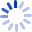# Regression Methods in Biostatistics: Linear, Logistic, Survival, and Repeated Measures Models

Here is a unified, readable introduction to multipredictor regression methods in biostatistics, including linear models for continuous outcomes, logistic models for binary outcomes, the Cox model for right-censored survival times, and generalized linear models for counts and other outcomes. The authors describe shared elements in methods for selecting, estimating, checking, and interpreting each model, and show that these regression methods deal with confounding, mediation, and interaction of causal effects in essentially ...

##### Cash for Textbooks